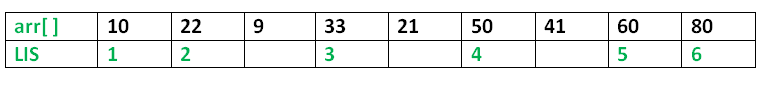Related Articles
C/C++ Program for Longest Increasing Subsequence
• Difficulty Level : Medium
• Last Updated : 04 Dec, 2018

The Longest Increasing Subsequence (LIS) problem is to find the length of the longest subsequence of a given sequence such that all elements of the subsequence are sorted in increasing order. For example, the length of LIS for {10, 22, 9, 33, 21, 50, 41, 60, 80} is 6 and LIS is {10, 22, 33, 50, 60, 80}.More Examples:

```Input  : arr[] = {3, 10, 2, 1, 20}
Output : Length of LIS = 3
The longest increasing subsequence is 3, 10, 20

Input  : arr[] = {3, 2}
Output : Length of LIS = 1
The longest increasing subsequences are {3} and {2}

Input : arr[] = {50, 3, 10, 7, 40, 80}
Output : Length of LIS = 4
The longest increasing subsequence is {3, 7, 40, 80}
```

Optimal Substructure:
Let arr[0..n-1] be the input array and L(i) be the length of the LIS ending at index i such that arr[i] is the last element of the LIS.
Then, L(i) can be recursively written as:
L(i) = 1 + max( L(j) ) where 0 < j < i and arr[j] < arr[i]; or
L(i) = 1, if no such j exists.
To find the LIS for a given array, we need to return max(L(i)) where 0 < i < n.
Thus, we see the LIS problem satisfies the optimal substructure property as the main problem can be solved using solutions to subproblems.

Following is a simple recursive implementation of the LIS problem. It follows the recursive structure discussed above.

 `/* A Naive C/C++ recursive implementation of LIS problem */` `#include ` `#include ` ` `  `/* To make use of recursive calls, this function must return ` `   ``two things: ` `   ``1) Length of LIS ending with element arr[n-1]. We use ` `      ``max_ending_here for this purpose ` `   ``2) Overall maximum as the LIS may end with an element ` `      ``before arr[n-1] max_ref is used this purpose. ` `   ``The value of LIS of full array of size n is stored in ` `   ``*max_ref which is our final result */` `int` `_lis(``int` `arr[], ``int` `n, ``int``* max_ref) ` `{ ` `    ``/* Base case */` `    ``if` `(n == 1) ` `        ``return` `1; ` ` `  `    ``// 'max_ending_here' is length of LIS ending with arr[n-1] ` `    ``int` `res, max_ending_here = 1; ` ` `  `    ``/* Recursively get all LIS ending with arr, arr ... ` `       ``arr[n-2]. If   arr[i-1] is smaller than arr[n-1], and ` `       ``max ending with arr[n-1] needs to be updated, then ` `       ``update it */` `    ``for` `(``int` `i = 1; i < n; i++) { ` `        ``res = _lis(arr, i, max_ref); ` `        ``if` `(arr[i - 1] < arr[n - 1] && res + 1 > max_ending_here) ` `            ``max_ending_here = res + 1; ` `    ``} ` ` `  `    ``// Compare max_ending_here with the overall max. And ` `    ``// update the overall max if needed ` `    ``if` `(*max_ref < max_ending_here) ` `        ``*max_ref = max_ending_here; ` ` `  `    ``// Return length of LIS ending with arr[n-1] ` `    ``return` `max_ending_here; ` `} ` ` `  `// The wrapper function for _lis() ` `int` `lis(``int` `arr[], ``int` `n) ` `{ ` `    ``// The max variable holds the result ` `    ``int` `max = 1; ` ` `  `    ``// The function _lis() stores its result in max ` `    ``_lis(arr, n, &max); ` ` `  `    ``// returns max ` `    ``return` `max; ` `} ` ` `  `/* Driver program to test above function */` `int` `main() ` `{ ` `    ``int` `arr[] = { 10, 22, 9, 33, 21, 50, 41, 60 }; ` `    ``int` `n = ``sizeof``(arr) / ``sizeof``(arr); ` `    ``printf``(``"Length of lis is %d\n"``, ` `           ``lis(arr, n)); ` `    ``return` `0; ` `} `

Output:

```Length of lis is 5
```

Please refer complete article on Dynamic Programming | Set 3 (Longest Increasing Subsequence) for more details!

Attention reader! Don’t stop learning now. Get hold of all the important DSA concepts with the DSA Self Paced Course at a student-friendly price and become industry ready.

My Personal Notes arrow_drop_up
Recommended Articles
Page :